# Big O Notation: A primer for beginning devs

Dec 26, 2019 - 9 min read
EducativeBig O notation is one of the most fundamental tools for computer scientists to analyze the time and space complexity of an algorithm.

Your choice of algorithm and data structure starts to matter when you’re tasked with writing software with strict SLAs (service level agreements) or for millions of users.

Big O Notation allows you to compare the efficiency of different algorithms, so when it comes to writing software, you can build a well designed and thought out application that will scale and perform better than competitors.

Here’s what will be covered:

## What is Big O Notation?

Big O Notation is a mathematical function used in computer science to describe how complex an algorithm is — or more specifically, the execution time required by an algorithm. In software engineering, it’s used to compare the efficiency of different approaches to a problem.

With Big O Notation, you express the runtime in terms of how quickly it grows relative to the input, as the input gets arbitrarily large. Essentially, it’s a way to draw insights into how scalable an algorithm is.

It doesn’t tell you how fast or how slow an algorithm will go, but instead about how it changes with respect to its input size.

## What are time and space complexity?

When talking about Big O Notation it’s important that you understand the concepts of time and space complexity, mainly because Big O Notation is a way to classify complexities.

Complexity is an approximate measure of the efficiency of an algorithm and is associated with every algorithm you write. This is something that all programmers must be aware of.

There are two kinds of complexities: time and space. Time complexity and space complexity are essentially approximations of how much time and how much space an algorithm will take to process certain inputs respectively.

Typically, there are three tiers to solve for (best case, average case, and worst case) which are known as asymptotic notations. These notations allow us to answer questions such as:

• Does the algorithm suddenly become incredibly slow when the input size grows?
• Does it mostly maintain its quick run time as the input size increases?

Best case — represented as Big Omega or Ω(n)

• Big-Omega, commonly written as Ω, is an Asymptotic Notation for the best case, or a floor growth rate for a given function. It provides us with an asymptotic lower bound for the growth rate of the runtime of an algorithm.

Average case — represented as Big Theta or Θ(n)

• Theta, commonly written as Θ, is an Asymptotic Notation to denote the asymptotically tight bound on the growth rate of runtime of an algorithm.

Worst case — represented as Big O Notation or O(n)

• Big-O, commonly written as O, is an Asymptotic Notation for the worst case, or ceiling of growth for a given function. It provides us with an asymptotic upper bound for the growth rate of the runtime of an algorithm.

Developers typically solve for the worst case scenario, Big O, because you’re not expecting your algorithm to run in the best or even average cases. It allows you to make analytical statements such as, “well, in the worst case scenario, my algorithm will scale this quickly”.

## Common varieties of Big O Notation

These varieties of Big-O Notation aren’t the only ones, but they’re the ones you’re most likely to encounter.

### O(1) - Constant time complexity

This translates to a constant runtime, meaning, regardless of the size of the input, the algorithm will have the same runtime.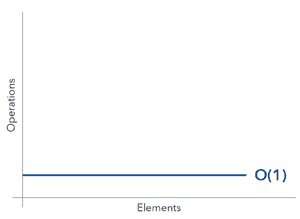Example:

Array: inserting or retrieving an element

### O(log n) - Logarithmic time complexity

O(log n) means that time goes up linearly, while the n goes up exponentially. So if it takes 1 second to compute 10 elements, it will take 2 seconds to compute 100 elements and so on. It is O(log n) when we use divide and conquer algorithms e.g binary search.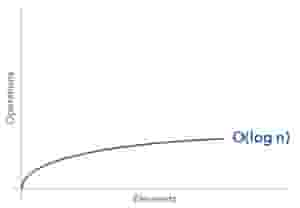Example:

Binary tree: inserting or retrieving an element

### O(n) - Linear time complexity

O(n) describes an algorithm whose performance will grow linearly and in direct proportion to the size of the input data set.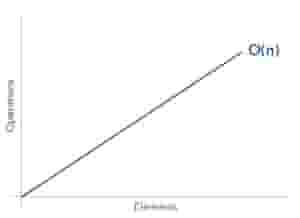Example:

Tree: Depth first search (DFS) of a tree

### O(n²) - Quadratic time complexity

As the elements in your list increase, the time it will take for your algorithm to run will increase exponentially.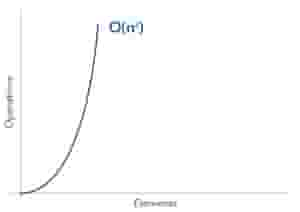Example:

Sorting algorithm: Bubble sort and insertion sort

#### Keep the learning going.

Learn Big O Notation without scrubbing through videos or documentation. Educative’s text-based courses are easy to skim and feature live coding environments — making learning quick and efficient.

Big O Notation for Coding Interviews and Beyond

## Asymptotic Analysis: How to find the complexity of an algorithm

### How to find Big O of an algorithm

If you’re in an interview and are asked to find the Big O complexity of an algorithm here is a general rule:

• Ignore the lower order terms

Example: Find the Big O complexity of an algorithm with the time complexity 3n³ + 4n + 2.

This simplifies to O(n³).

### How to find the time complexity of an algorithm

There are three steps you’ll want to take when calculating the time complexity of an algorithm:

• List down all the basic operations in the code
• Count the number of times each gets executed
• Sum all the counts to get an equation

Here’s a simple example that measures the time complexity of a for loop of size “n”:

#include <iostream>
using namespace std;
int main(){
int n = 10;  //  0(1)
int sum = 0; // 0(1)
for (int i=0; i<n; i++)
sum+=2; // 0(1)
cout << sum; // 0(1)
return 0;
}


First, split the code into individual operations and then compute how many times it is being executed, which will look like this:

Operation Number of Executions
int n = 10; 1
int sum = 0; 1
int i = 0; 1
i < n; n + 1
i++; n
sum += 2; n
cout << sum; 1

After counting the number of operations and how many times each operation is executing, you just add all of these counts to get the time complexity of this program.

Time Complexity = $1 + 1 + 1 + (n + 1) + n + n + 1 = 3 + (n + 1) + 2n + 1 => 3n + 5$

General tips for asymptotic analysis:

• Every time a list or array gets iterated over c x length times, it is most likely in O(n) time.
• When you see a problem where the number of elements in the problem space gets halved each time, it will most probably be in O(logn) runtime.
• Whenever you have a singly nested loop, the problem is most likely in quadratic time.

Useful Formulae for calculating time complexity of an algorithm: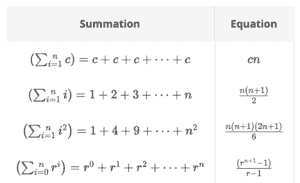## Big O Notation examples

### O(1)

 void printFirstItem(const vector<int>& items)
{
cout << items << endl;
}


This function runs in O(1) time (or “constant time”) relative to its input. This means that the input array could be 1 item or 1,000 items, but this function would still just require one “step.”

### O(n)

void printAllItems(const vector<int>& items)
{
for (int item : items) {
cout << item << endl;
}
}


This function runs in O(n) time (or “linear time”), where n is the number of items in the vector. If the vector has 10 items, we have to print 10 times. If it has 1,000 items, we have to print 1,000 times.

### O(n²)

void printAllPossibleOrderedPairs(const vector<int>& items)
{
for (int firstItem : items) {
for (int secondItem : items) {
cout << firstItem << ", " << secondItem << endl;
}
}
}


Here we’re nesting two loops. If our vector has n items, our outer loop runs n times and our inner loop runs n times for each iteration of the outer loop, giving us n^2 total prints. Thus this function runs in O(n^2) time (or “quadratic time”). If the vector has 10 items, we have to print 100 times. If it has 1,000 items, we have to print 1,000,000 times.

## Big O Notation cheat sheet

Data Structure Worst Case Notes
Array Insert: O(1) Retrieve: O(1) N/A
Linked List Insert at tail: O(n)
Retrieve: O(n)
Note that if new elements are added at the head of the linked list then insert becomes a O(1) operation
Binary Tree Insert: O(n)
Retrieve: O(n)
In worst case, the binary tree becomes a linked-list.
Dynamic Array Insert: O(1)
Retrieve: O(1)
Note by retrieving it is implied we are retrieving from a specific index of the array.
Stack Push: O(1)
Pop: O(1)
There are no complexity trick questions asked for stacks or queues. We only mention them here for completeness. The two data-structures are more important from a last-in last-out (stack) and first in first out (queue) perspective.
Queue Enqueue: O(1)
Dequeue: O(1)
N/A
Priority Queue (Binary Heap) Insert: O(logn)
Delete: O(logn)
Get Min/Max: O(1)
N/A
Hash Table Insert: O(n)
Retrieve: O(n)
Be mindful that a hash table’s average case for insertion and retrieval is O(1)
B-Trees Insert: O(logn)
Retrieve: O(logn)
N/A
Red-Black Trees Insert: O(logn)
Retrieve: O(logn)
N/A

Algorithm Worst Case Notes
Sorting / Searching Algorithm Bubble sort: O(n²)
Insertion sort: O(n²)
Selection sort: O(n²)
Quick sort: O(n²)
Merge sort: O(logn)
Note, even though worst case quicksort performance is O(n2) but in practice quicksort is often used for sorting since its average case is O(nlgn).
Tree Algorithm Depth first search: O(n)
Pre-order, in-order, post-order traversals: O(n)
n is the total number of nodes in the tree. Most tree-traversal algorithms will end up seeing every node in the tree and their complexity in the worst case is thus O(n).

## Where to go from here

While there was quite a bit covered in this post, we’ve barely even scratched the surface.

Big O For Coding Interviews & Beyond is a simple and practical guide to algorithmic complexity. Learn what you need to know specifically to analyze any algorithm, and ace your next coding interview.

This course is designed for folks who aren’t math whizzes, or even super-experienced in programming. Written in a conversational style, chock full of real-world examples, this course is an antidote to the mountains of dry, technical Big-O references.

By the time you wrap up this course, you’ll have a working knowledge of Big-O, and the confidence to ace your programming interview at any tech company!